Courses

# Transistor As an Amplifier Notes | EduRev

## Electrical Engineering (EE) : Transistor As an Amplifier Notes | EduRev

The document Transistor As an Amplifier Notes | EduRev is a part of the Electrical Engineering (EE) Course Analog Electronics.
All you need of Electrical Engineering (EE) at this link: Electrical Engineering (EE)

TRANSISTOR AS AN AMPLIFIER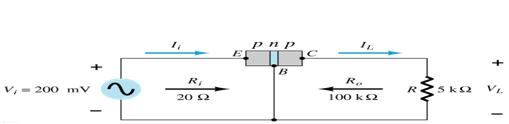Common-Emitter Configuration

It is called common-emitter configuration since : emitter is common or reference to both input and output terminals. Emitter is usually the terminal closest to or at ground potential.

Amplifier design is using connection of CE due to the high gain for current and voltage.

Two set of characteristics are necessary to describe the behavior for CE i.e. input (base terminal) and output (collector terminal) parameters.

Proper Biasing of common-emitter configuration in active region.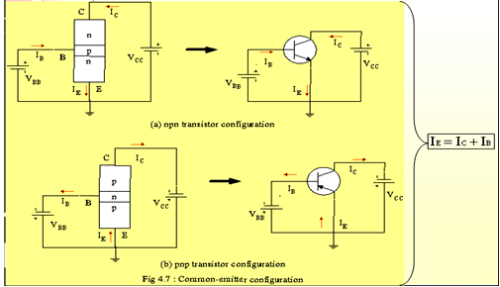IB is in microamperes compared to milliamperes of IC.

IB will flow when VBE > 0.7V for silicon and 0.3V for germanium.

Before this value, IB is very small.

Base-emitter junction is forward bias. Increasing VCE will reduce IB for different values.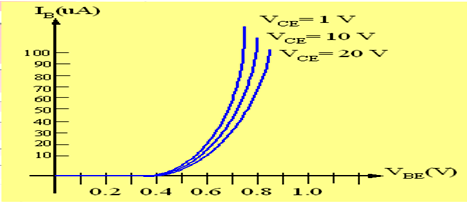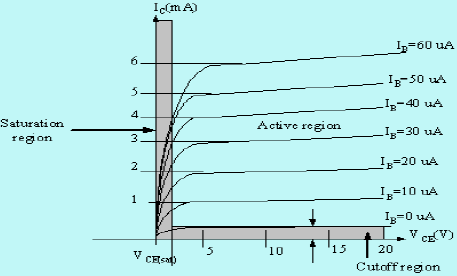Output characteristics for common-emitter npn transistor.

For small value of VCE (VCE < VCESAT, IC increases linearly with increasing value of VCE

and if  VCE > VCESAT, IC does not totally depend on VCE → constant IC

IB(µA) is very small compared to IC (mA). Small increase in IB causes big increase in IC

IB=0A → ICEO occurs.

Noticing the value when IC= 0A. There is still some value of current flowing.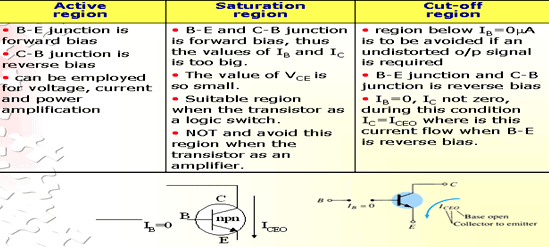Beta (β) or amplification factor

The ratio of dc collector current (IC) to the dc base current (IB) is dc beta (βdc ) which is dc current gain where IC and IB are determined at a particular operating point, Q-point (quiescent point).  It is defined by the following equation:

30 < βdc < 300 → 2N3904   (IC no.)

On data sheet, βdc=hfe where h is derived from ac hybrid equivalent circuit. FE are derived from forward-current amplification and common-emitter configuration respectively.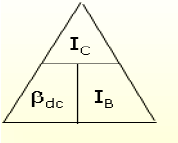For ac conditions, an ac beta has been defined as the changes of collector current (IC) compared to the changes of base current (IB) where IC and IB are determined at the operating point. On data sheet, βac=hfe  It can defined by the following equation: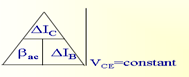From output characteristics of common emitter configuration, find βac and βdc with an

Operating point at IB=25 mA and VCE =7.5V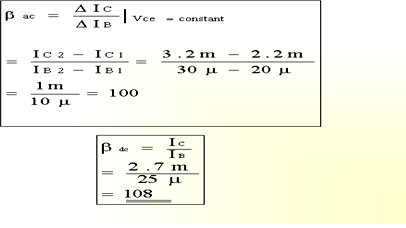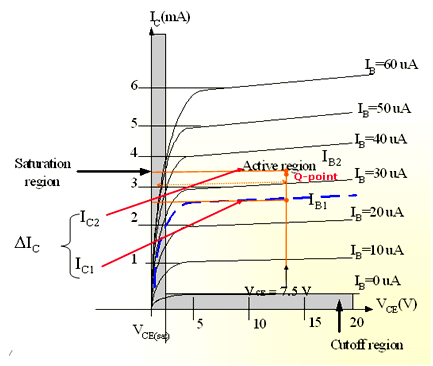Relationship analysis between α and β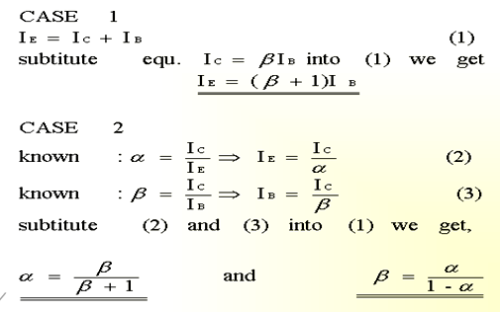Offer running on EduRev: Apply code STAYHOME200 to get INR 200 off on our premium plan EduRev Infinity!

## Analog Electronics

11 videos|56 docs|97 tests

,

,

,

,

,

,

,

,

,

,

,

,

,

,

,

,

,

,

,

,

,

;